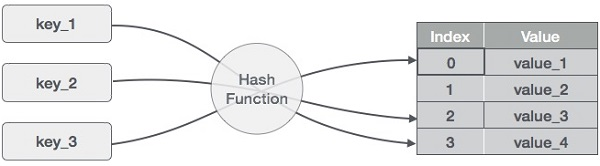Options
All
• Public
• Public/Protected
• All

# Class Dictionary<T>

## Dictionary (hash table)

In computing, a hash table (hash map) is a data structure that implements an associative array abstract data type, a structure that can map keys to values. A hash table uses a hash function to compute an index, also called a hash code, into an array of buckets or slots, from which the desired value can be found. Ideally, the hash function will assign each key to a unique bucket, but most hash table designs employ an imperfect hash function, which might cause hash collisions where the hash function generates the same index for more than one key. Such collisions are always accommodated in some way.source: tutorialspoint.com

### Complexity

Algorithm Average Worst case
Access `O(1)` `O(n)`
Search (by key) `O(1)` `O(n)`
Search (by value) `O(n)` `O(n)`
Insert `O(1)` `O(n)`
Delete `O(1)` `O(n)`
- - -
Space `O(n)` `O(n)`

### Reference

``````import { Dictionary } from '@pencroff/ts-algorithms/dist/structure/dictionary';
const q = new Dictionary([1, 2, 3])``````

Dictionary used LinkedList for buckets and murmur2_32 as hashing function (selected as most randomness - link).

At the moment Dictionary keys expected to be `string`

#### Note:

Native JS object is kind of Dictionary

• Dictionary

### Implements

• Iterable<[string, T]>

## Constructors

### constructor

• Dictionary constructor

#### Parameters

• ##### Optional initArr: [string, T][]

initial data as array of tuples - [key, value]

• ##### Optional sizeOptions: number | DictionaryOptions<T>

initial Dictionary size or DictionaryOptions object

## Properties

_len: number

### Private options

options: DictionaryOptions<T>

## Accessors

### length

• get length(): number

### size

• get size(): number

## Methods

### __@iterator

• __@iterator(): Iterator<[string, T]>
• Dictionary iterator for iteration across all key-value tuples

Complexity: O(n)

### clear

• clear(): void

### get

• get(key: string): T
• Get value from Dictionary by key

#### Parameters

• ##### key: string

Complexity: O(1) / O(n)

### Private getNewSize

• getNewSize(oldSize: number): number

### Private getSize

#### Returns number

• Check if Dictionary has a `key`

#### Parameters

• ##### key: string

Complexity: O(1) / O(n)

### hasValue

• hasValue(value: T): boolean
• Check if Dictionary has a `value`

#### Parameters

• ##### value: T

Complexity: O(n)

### keys

• keys(): Iterable<string>
• Dictionary iterator for iteration across all keys

Complexity: O(n)

### remove

• remove(key: string): boolean
• Remove value from Dictionary by key

#### Returns boolean

`true` if key-value was really deleted, `false` if no key available

Complexity: O(1) / O(n)

### resize

• resize(): boolean
• Resize dictionary for most efficient data access Called automatically, if length equal size, before insert new key-value pair Increased size 25% of prev value, 100% or 50% for size less then 32

Complexity: O(n)

### set

• set(key: string, value: T): void
• Set / update value in Dictionary by key

#### Parameters

• ##### value: T

Complexity: O(1) / O(n)

### values

• values(): Iterable<T>
• Dictionary iterator for iteration across all values

Complexity: O(n)